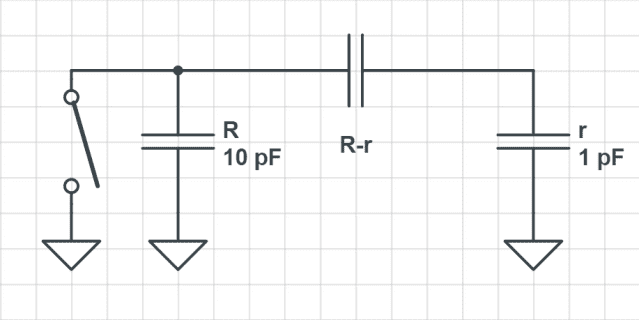# Electrostatic induction in different size spheres

• abdo799

#### abdo799

if we have a charged sphere with charge Q and radius r, the voltage on it's surface is calculated by gauss's law, what if we approach to it another sphere with radius R , R>r, Earth it, remove earth, basically charging by induction, will the sphere have the same charge, which means less voltage on the surface or same voltage which means more charge?
In other words my question is , which one is induced the voltage or the charge?

You can model this situation with an equivalent circuit diagram.R and r both have a capacity relative to ground and they also have a capacity relative to each other. CRr is variable and depends on the distance of the two spheres to each other. So first you bring R and r very close, which makes CRr large. Then you ground R (i.e. you close the switch) for a moment which gives CRr about the same amount of charge as Cr assuming that CRr is much larger than the capacity of the two spheres. After you open the switch again you move the two spheres apart i.e. make CRr small. As a result all of the charge from CRr is dumped into R which will then have about the same amount of charge as r but with opposite polarity. Of course if CRr is not that large because the two spheres are not close enough then R will and up with less charge than r.
Btw. The voltage that drops over CR while it is still uncharged i.e. before you close the switch, will get closer and closer to the voltage of Cr as the two spheres approach each other but will not exceed it. Only after you ground R and then remove it from r can it's voltage become higher than that of r and that only if R is smaller than r.

Last edited: